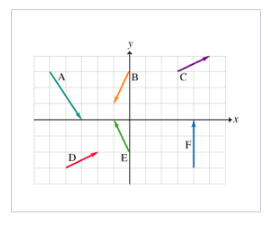# Problem: Six vectors ( A to F) have the magnitudes and directions indicated in the figure. Which two vectors, when added, will have the largest (positive) y component?1. C &amp; D2. A &amp; F3. E &amp; F4. A &amp; B5. E &amp; D

###### FREE Expert Solution

A = 2i - 3j

B = -1i - 2j

C = 2i + 1j

D = 2i + 1j

E = -1i + 2j

F = 0 i + 3j

Sum of y-components:

80% (345 ratings)###### Problem Details

Six vectors ( A to F) have the magnitudes and directions indicated in the figure.Which two vectors, when added, will have the largest (positive) y component?

1. C & D

2. A & F

3. E & F

4. A & B

5. E & D